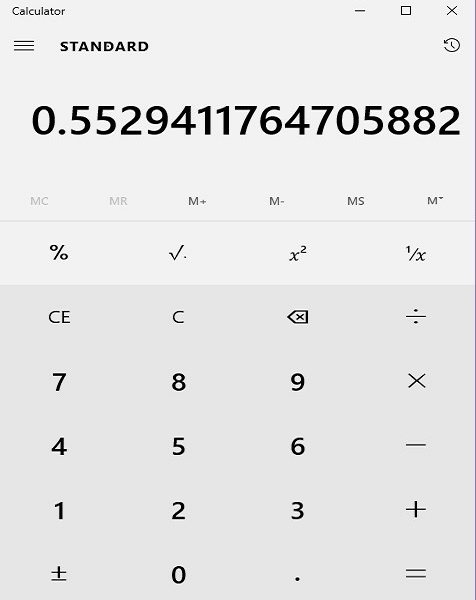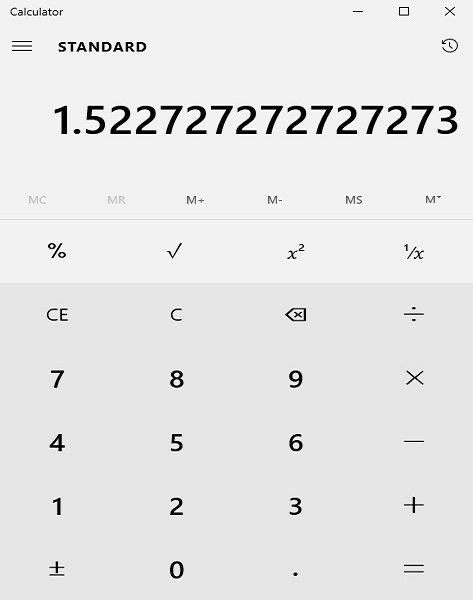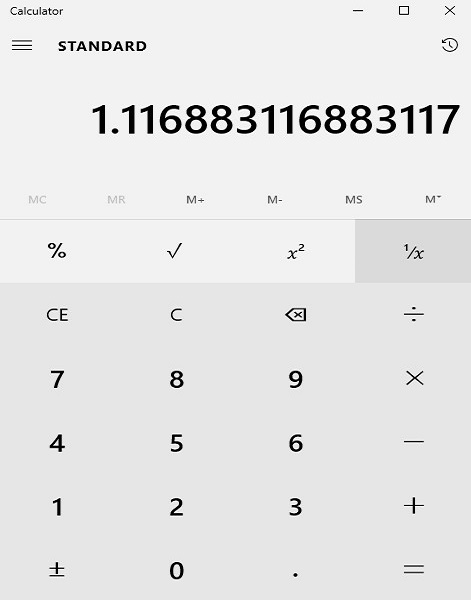# Using a Calculator to Convert a Fraction to a Rounded Decimal

#### Complete Python Prime Pack

9 Courses     2 eBooks

#### Artificial Intelligence & Machine Learning Prime Pack

6 Courses     1 eBooks

#### Java Prime Pack

9 Courses     2 eBooks

An electronic calculator displays fractions as decimal approximations rounded up to certain number (8, 12 or any other number) of places of decimals depending upon the company, type and model.

In this lesson, we learn how to use a calculator to convert a fraction to a rounded decimal.

Suppose we want the value of a fraction to be rounded to n places of decimal. We take the value with first n+1 digits after the decimal point from the calculator and round to n places i.e., we take one more digit than the required number of places of decimals. Then we round off the last digit. If the last digit is 5 or more, we add 1 to the preceding digit and if 4 or less we drop the last digit and write the first four digits as it is.

For example, suppose we want the value of a fraction to be rounded to four places of decimal. We take the value with first five digits after the decimal point from the calculator and round to four places i.e., we take one more digit than the required number of places of decimals. Then we round off the last digit. If the last digit is 5 or more, we add 1 to the preceding digit and if 4 or less we drop the last digit and write the first four digits as it is.

Using a calculator convert $\frac{47}{85}$ into a decimal rounded to four places of decimal.

### SolutionStep 1:

At first, we set up the fraction $\frac{47}{85}$ as a long division problem, dividing 47 by 85 using a calculator.

Step 2:

We find that on long division $\frac{47}{85} = 0.55294117...$

Step 3:

Since we must round to four places of decimal, we consider the first five (one more than four) digits after the decimal, i.e., 55294

Step 4:

The last digit is 4, so we drop it and keep the first four digits after the decimal only, i.e., 5529

Step 5:

So, $\frac{47}{85} = 0.5529$

Using a calculator convert $\frac{67}{44}$ into a decimal rounded to four places of decimal.

### SolutionStep 1:

At first, we set up the fraction as a long division problem, dividing 67 by 44

Step 2:

We find that on long division $\frac{67}{44} = 1.522727272...$

Step 3:

Since we must round to four places of decimal, we consider the first five (one more than four) digits after the decimal, i.e., 52272

Step 4:

The last digit is 2, so we drop it and keep the first four digits after the decimal only, i.e., 5227

Step 5:

So, $\frac{67}{44} = 1.5227$

Using a calculator convert $\frac{86}{77}$ into a decimal rounded to four places of decimal.

### SolutionStep 1:

At first, we set up the fraction as a long division problem, dividing 86 by 77

Step 2:

We find that on long division $\frac{86}{77} = 1.11688311...$

Step 3:

Since we must round to four places of decimal, we consider the first five (one more than four) digits after the decimal, i.e., 11688

Step 4:

The last digit is 8, so we add 1 to preceding digit which is also 8 to make it 9 and therefore rounding leads to, 1169

Step 5:

So, $\frac{86}{77} = 1.1169$